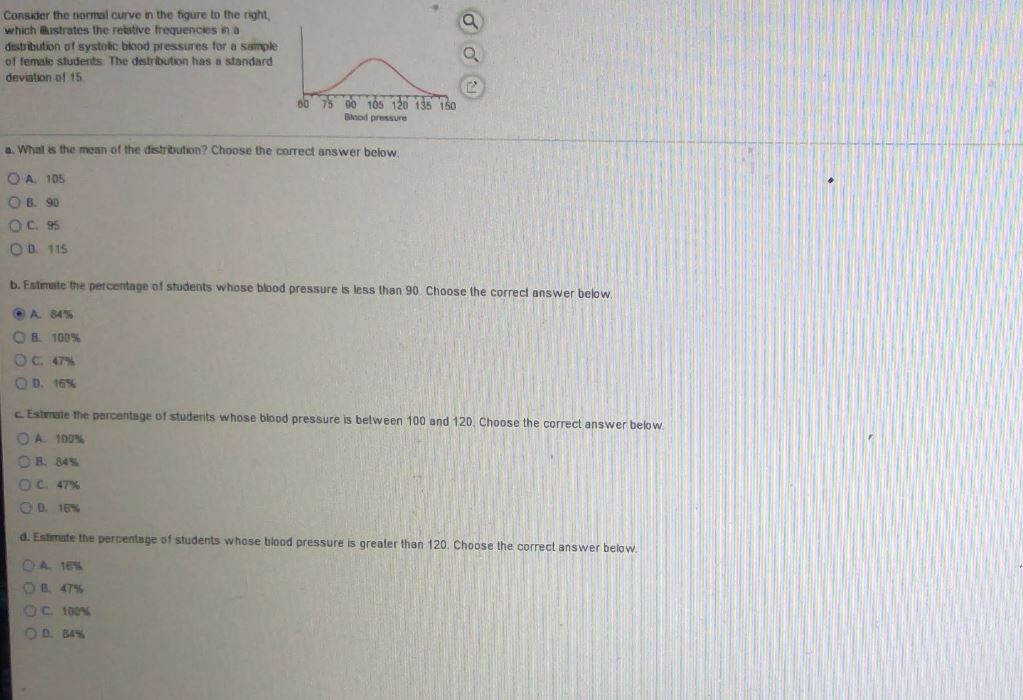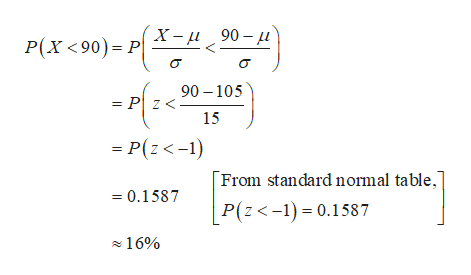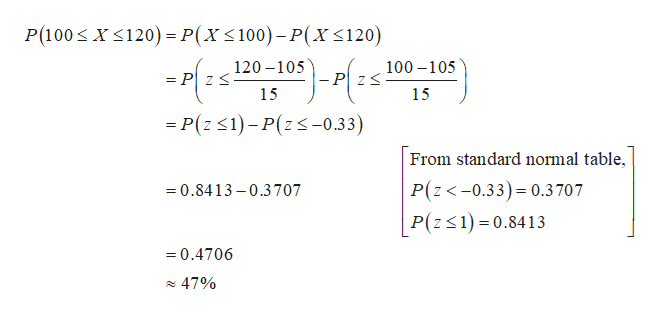# Consider the normal curve in the figure to the rightwhich ustrates the relative frequencies in adistribution of systolc biood pressures for a sampleof female students The destribution has a standarddevation of 1560 75 00 105 120 135 150Blood pressurea. What is the mean of the destribution? Choose the correct answer belowOA 105OB 90OC 95OD 115b. Estimate the percentage of students whose blood pressure is less than 90 Choose the correct answer belowA 84%OB 100%O c. 47%OD. 16%CEstmate the percentage of students whose blood pressure is between 100 and 120, Choose the correct answer belowOA 100%OR 84%OC 47%OD. 16%d. Estimate the percentage of students whose blood pressure is greater than 120. Choose the correct answer below.OA 16%OB 47%OC 100%OP 84%

Question
38 viewshelp_outlineImage TranscriptioncloseConsider the normal curve in the figure to the right which ustrates the relative frequencies in a distribution of systolc biood pressures for a sample of female students The destribution has a standard devation of 15 60 75 00 105 120 135 150 Blood pressure a. What is the mean of the destribution? Choose the correct answer below OA 105 OB 90 OC 95 OD 115 b. Estimate the percentage of students whose blood pressure is less than 90 Choose the correct answer below A 84% OB 100% O c. 47% OD. 16% CEstmate the percentage of students whose blood pressure is between 100 and 120, Choose the correct answer below OA 100% OR 84% OC 47% OD. 16% d. Estimate the percentage of students whose blood pressure is greater than 120. Choose the correct answer below. OA 16% OB 47% OC 100% OP 84% fullscreen
check_circle

Step 1

a.

The mean of the distribution is 105 and it is obtained below:

The mean of the normal distribution represents the middle value in the distribution plot. In the given distribution plot, the middle value is 105. Hence, the mean of the distribution is 105.

Thus, the correct option is A. 105.

Step 2

b.

The percentage of students whose blood pressure is less than 90 is 16% and it is calculated below:

The correct option is D. 16%.

From the given information, let us define the random variable X as the blood pressure of students follows normal distribution with a mean 105 and standard deviation 15.help_outlineImage TranscriptioncloseX-90- P(X<90) P 90 105 = P z< 15 = P(z<-1) From standard normal table, 0.1587 P(z<-1)0.1587 16% fullscreen
Step 3

c.

The percentage of students whose blood pressure is between 100 and 120 is 47% and it is calculated b...help_outlineImage TranscriptioncloseXS120)=P(X< 100)- P(X<120) P(100 120-105 100-105 = P ZS - P Z S 15 15 -P(z 1)-P(z-0.33) From standard normal table, P(z<-0.33) 0.3707 P(z1) 0.8413 0.8413-0.3707 =0.4706 47% fullscreen

### Want to see the full answer?

See Solution

#### Want to see this answer and more?

Solutions are written by subject experts who are available 24/7. Questions are typically answered within 1 hour.*

See Solution
*Response times may vary by subject and question.
Tagged in

### Statistics